# Download a FREE 3D Printing Lesson Plan for the SKETCH Classroom

In this 3D Printing Lesson Plan, students will verify (A+B)³ as a mathematical expression based on physical measurement through the use of modeling and 3D printing.

Lesson Plan: Math Expressions (A+B)³
Creator: Venkateswaran
Subject: Math
Level of Difficulty: Easy

This lesson plan is featured with the MakerBot SKETCH Classroom. With the SKETCH Classroom, students get more access to 3D printing and teachers are set up for success. This offering goes beyond the hardware and incorporates MakerBot's complete education ecosystem, providing educators and students with the tools and resources they need to succeed. Learn more about the MakerBot SKETCH Classroom here.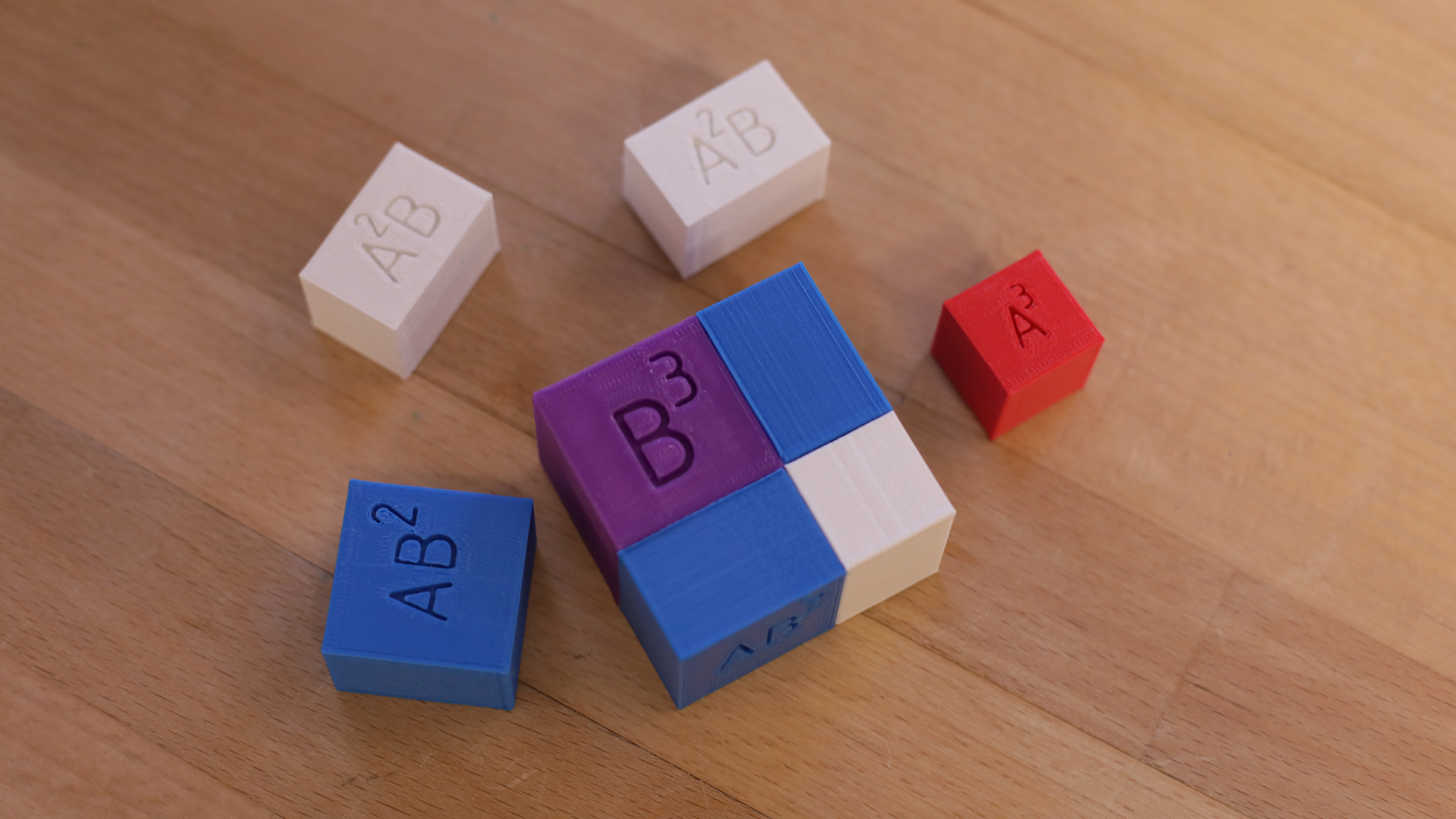#### STANDARDS COVERED

MS-ETS1-3 ENGINEERING DESIGN
Analyze data from tests to determine similarities and differences among several design solutions to identify the best
characteristics of each that can be combined into a new solution to better meet the criteria for success.

HS-ETS1-4 ENGINEERING DESIGN
Use a computer simulation to model the impact of proposed solutions to a complex real-world problem with numerous
criteria and constraints on interactions within and between systems relevant to the problem.

CCSS.MATH.CONTENT.7.EE.A.1
Apply properties of operations as strategies to add, subtract, factor, and expand linear expressions with rational coefficients.

#### TEACHER INSTRUCTIONS

TinkerCAD software was used to design the cubes. The dimensions are provided in the images for reference. The side A
was chosen to be 2cm and B was chosen to be 3cm. Rest of the calculations follow as per the equation:

(A+B)3 = A3+3A2B+3B2A+B3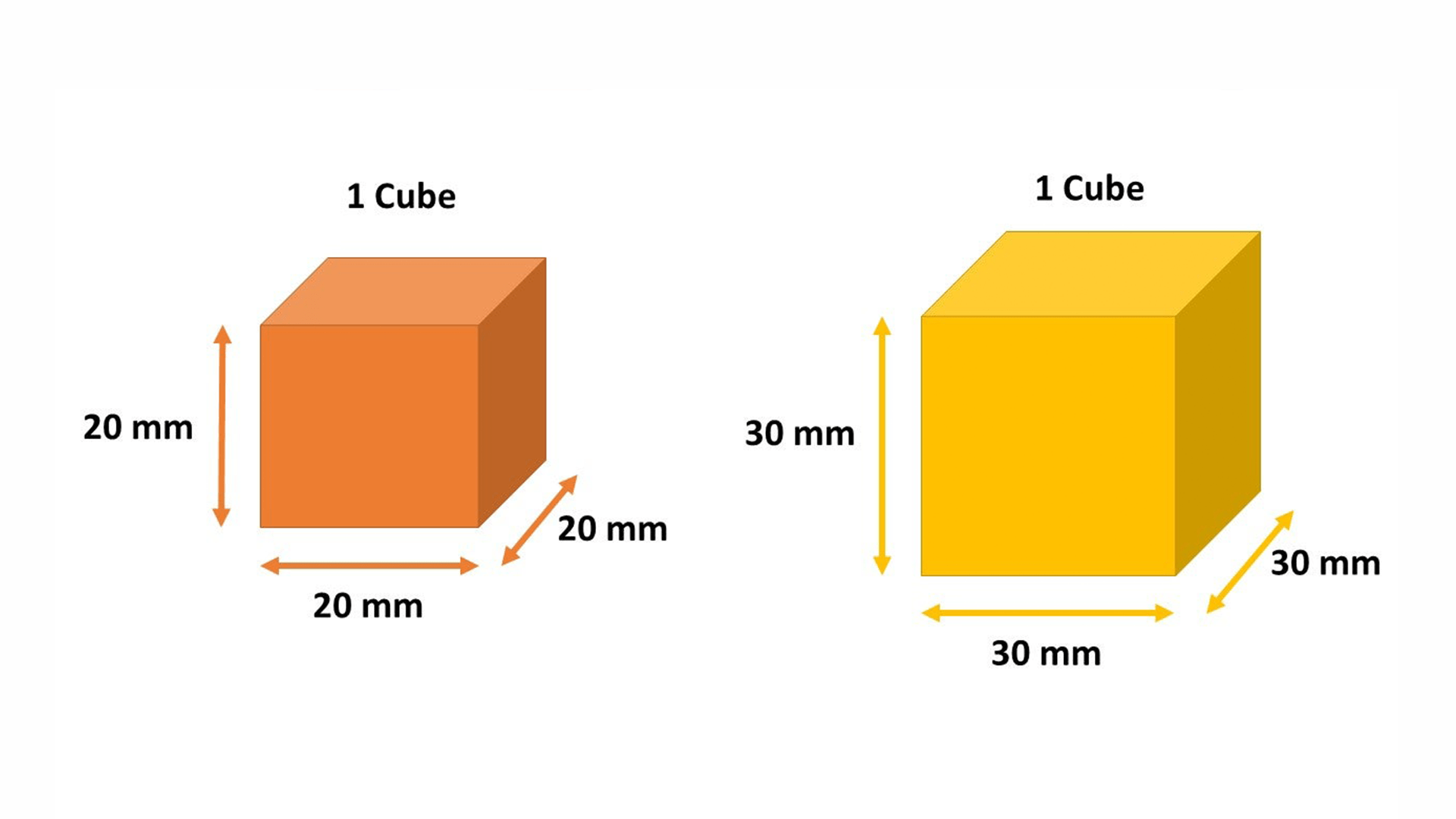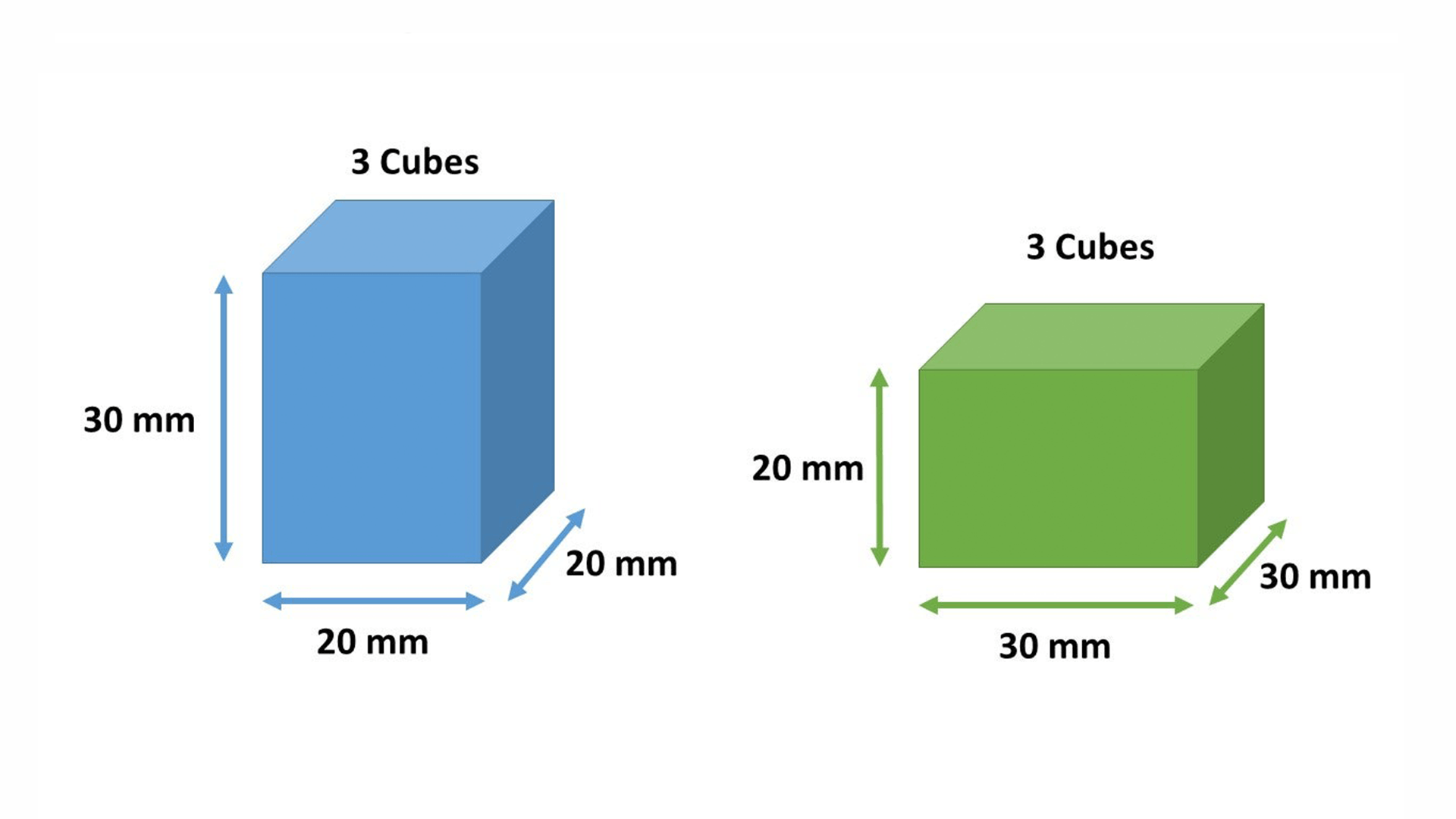#### STUDENT INSTRUCTIONS

Theoretical Explanation of the Math Expression with 3D Models
A3 and B3 Model Dimensions A2B and B2A Model Dimensions

Project Info:
Apply the knowledge of modeling primitive shapes using TinkerCAD
● Utilize physical measurement and modeling technique to other complex mathematical expressions and formulae
● Explain the concept of Pascal’s Triangle in an intuitive and novel approach
● Make use of technology to simplify the teaching approach for better and quicker understanding amongst students

Pascals Triangle:

1
1 1
1 2 1
1 3 3 1
1 4 6 4 1
1 5 10 10 5 1
1 6 15 20 15 6 1
...

For example:

(a+b)0 = 1
(a+b)1 = a1+b1 = a+b
(a+b)2 = a2+2ab+b2
(a+b)3 = a3+3a2b+3ab2+b3
(a+b)4 = a4+4a3b+6a2b2+4ab3+b4

...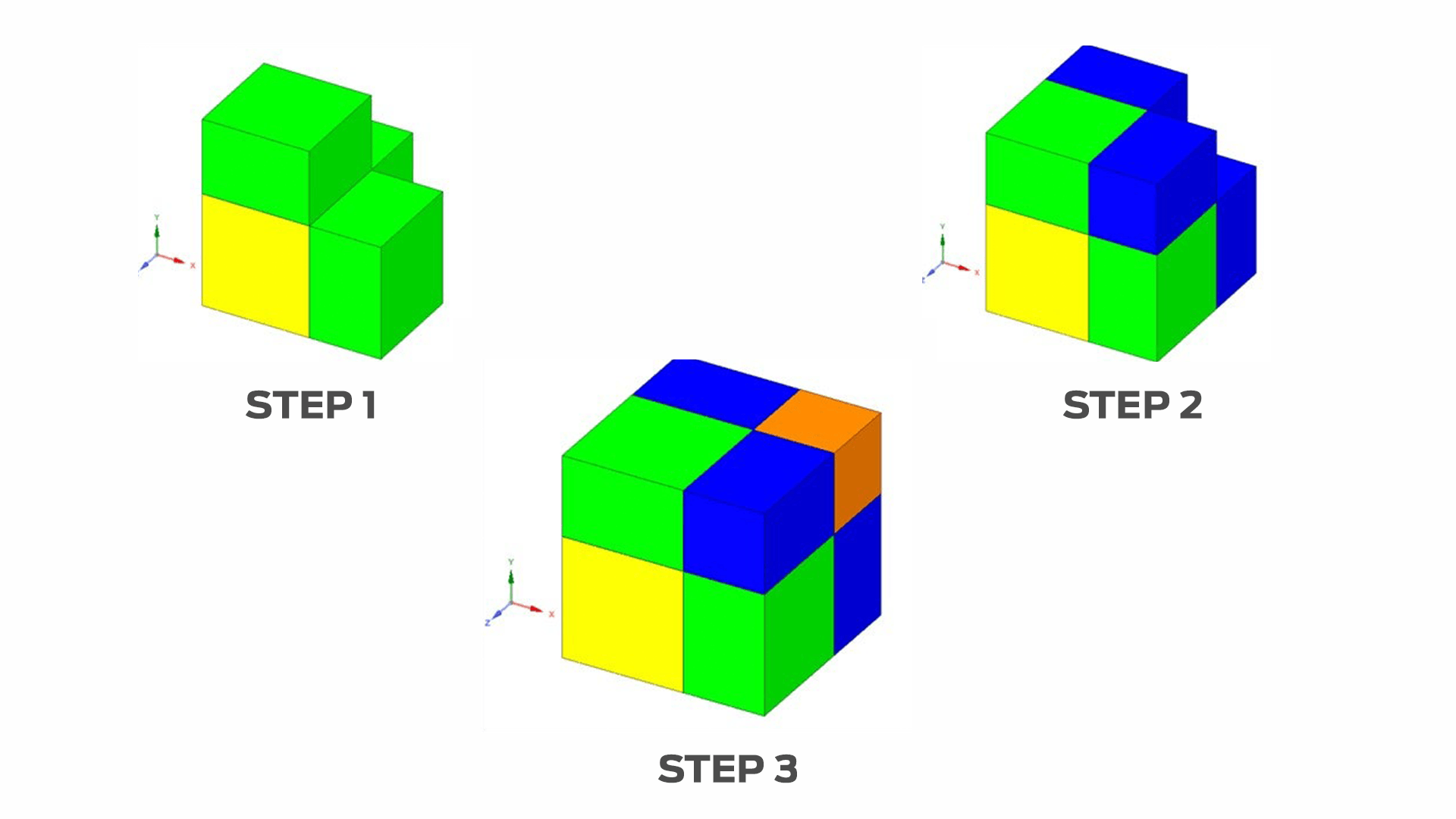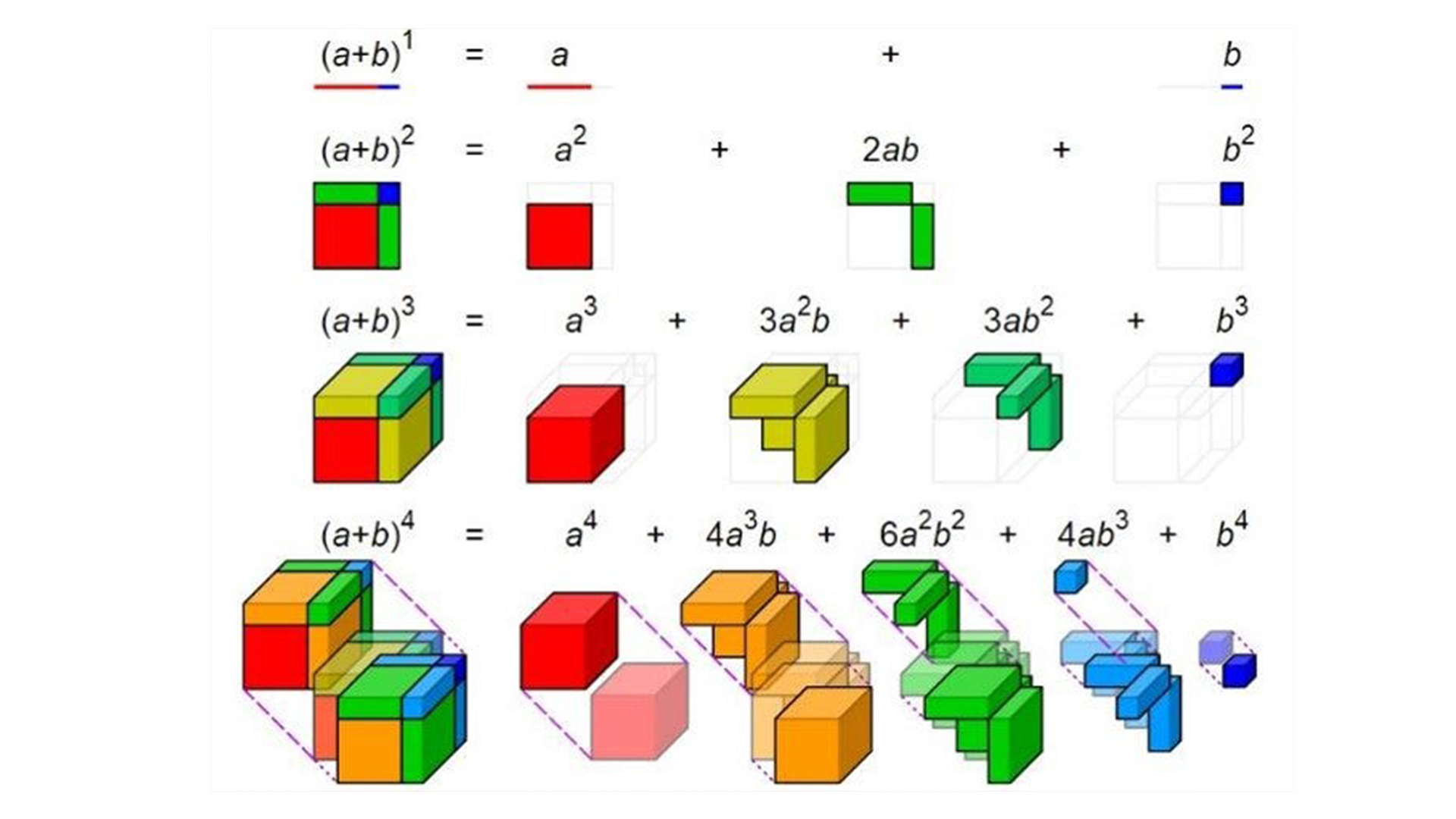#### KEY QUESTIONS

● How will you apply this learning session in your classroom?
● What are the subject areas in Mathematics and Physical Measurement wherein this modus of operand will help the
students grasp the concept?
● How will this technique help the students to apply it to their real life problems and applications?

#### AUTHOR NOTES

For a group of 30 Students, you may have to split them into a batch of 5 students each. So for each batch you would need one set of cubes without names engraved on them and one more set of cubes with name engraved in them. During the first 75% of the teaching pedagogy you would need only the cubes without the names engraved.

After the printing of a cube is initiated, you may collect the cubes (without names) back and then hand over the cubes with names and teach them the mathematical expression and ask them to assemble.

The A2B and B2A models need to printed in quantities of 3 each. The A3 and B3 models need one quantity each. Also the A2B and B2A models need to printed in quantities of 3 each. The A3 and B3 models need one quantity each.

**Remember in order to print them in different colors, you will have to load them separately.

<